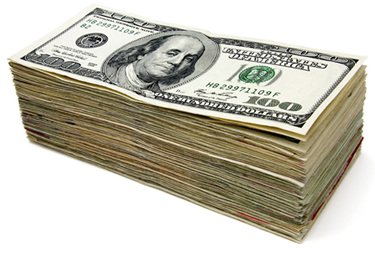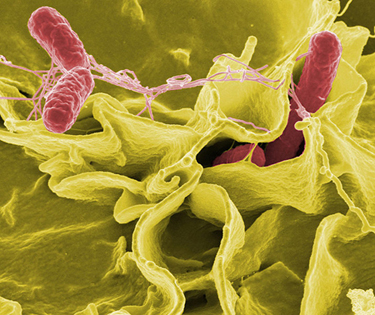Chapter 1 The Nature of Science and Physics

5 1.4 Approximation

Summary

• Make reasonable approximations based on given data.

On many occasions, physicists, other scientists, and engineers need to make approximations or “guesstimates” for a particular quantity. What is the distance to a certain destination? What is the approximate density of a given item? About how large a current will there be in a circuit? Many approximate numbers are based on formulae in which the input quantities are known only to a limited accuracy. As you develop problem-solving skills (that can be applied to a variety of fields through a study of physics), you will also develop skills at approximating. You will develop these skills through thinking more quantitatively, and by being willing to take risks. As with any endeavor, experience helps, as well as familiarity with units. These approximations allow us to rule out certain scenarios or unrealistic numbers. Approximations also allow us to challenge others and guide us in our approaches to our scientific world. Let us do two examples to illustrate this concept.

Example 1: Approximate the Height of a Building

Can you approximate the height of one of the buildings on your campus, or in your neighborhood? Let us make an approximation based upon the height of a person. In this example, we will calculate the height of a 39-story building.

Strategy

Think about the average height of an adult male. We can approximate the height of the building by scaling up from the height of a person.

Solution

Based on information in the example, we know there are 39 stories in the building. If we use the fact that the height of one story is approximately equal to about the length of two adult humans (each human is about 2-m tall), then we can estimate the total height of the building to be

$\boldsymbol{\frac{2\textbf{ m}}{1\;\textbf{person}}}$ $\boldsymbol{\times}$ $\boldsymbol{\frac{2 \;\textbf{person}}{1\;\textbf{story}}}$ $\boldsymbol{\times}$ $\boldsymbol{39 \;\textbf{stories}=156 \;\textbf{m}}$

Discussion

You can use known quantities to determine an approximate measurement of unknown quantities. If your hand measures 10 cm across, how many hand lengths equal the width of your desk? What other measurements can you approximate besides length?

Example 2: Approximating Vast Numbers: a Trillion DollarsFigure 1. A bank stack contains one-hundred $100 bills, and is worth$10,000. How many bank stacks make up a trillion dollars? (credit: Andrew Magill).

The U.S. federal deficit in the 2008 fiscal year was a little greater than $10 trillion. Most of us do not have any concept of how much even one trillion actually is. Suppose that you were given a trillion dollars in$100 bills. If you made 100-bill stacks and used them to evenly cover a football field (between the end zones), make an approximation of how high the money pile would become. (We will use feet/inches rather than meters here because football fields are measured in yards.) One of your friends says 3 in., while another says 10 ft. What do you think?

Strategy

When you imagine the situation, you probably envision thousands of small stacks of 100 wrapped \$100 bills, such as you might see in movies or at a bank. Since this is an easy-to-approximate quantity, let us start there. We can find the volume of a stack of 100 bills, find out how many stacks make up one trillion dollars, and then set this volume equal to the area of the football field multiplied by the unknown height.

Solution

(1) Calculate the volume of a stack of 100 bills. The dimensions of a single bill are approximately 3 in. by 6 in. A stack of 100 of these is about 0.5 in. thick. So the total volume of a stack of 100 bills is:

$\begin{array}{lcl} \textbf{volume of stack} & = & \textbf{length}\times\textbf{width}\times\textbf{height,} \\ \textbf{volume of stack} & = & \boldsymbol{6}\textbf{ in.}\times\boldsymbol{3}\textbf{ in.}\times\boldsymbol{0.5}\textbf{ in.,} \\ \textbf{volume of stack} & = & \boldsymbol{9\textbf{ in.}^3.}\end{array}$

(2) Calculate the number of stacks. Note that a trillion dollars is equal to $\boldsymbol{\1\times10^{12}}$, and a stack of one-hundred $\boldsymbol{\100}$ bills is equal to $\boldsymbol{\10,000}$, or $\boldsymbol{\1\times10^4}$. The number of stacks you will have is:

$\boldsymbol{\1\times10^{12}\textbf{(a trillion dollars)} / \1\times10^4\textbf{ per stack}=1\times10^8\textbf{ stacks.}}$

(3) Calculate the area of a football field in square inches. The area of a football field is $\boldsymbol{100\textbf{ yd}\times50\textbf{ yd},}$ which gives $\boldsymbol{5000\textbf{ yd}^2}$ Because we are working in inches, we need to convert square yards to square inches:

$\boldsymbol{\textbf{Area}=5000\textbf{ yd}^2\times\frac{3\textbf{ ft}}{1\textbf{ yd}}\times\frac{3\textbf{ ft}}{1\textbf{ yd}}\times\frac{12\textbf{ in.}}{1\textbf{ ft.}}\times\frac{12\textbf{ in.}}{1\text{ ft.}}=6,480,000\textbf{ in.}^2}$
$\boldsymbol{\textbf{Area}\approx{6\times10^6\textbf{ in.}^2}}$

This conversion gives us $\boldsymbol{6\times10^6\textbf{ in.}^2}$ for the area of the field. (Note that we are using only one significant figure in these calculations.)

(4) Calculate the total volume of the bills. The volume of all the$\boldsymbol{\100\textbf{-bill}}$ stacks is
$\boldsymbol{9\textbf{ in.}^3/\textbf{stack}\times10^8\textbf{ stacks}=9\times10^8\textbf{ in.}^3}$.

(5) Calculate the height. To determine the height of the bills, use the equation:

$\begin{array}{lcl} \textbf{volume of bills} & = & \textbf{area of field }\times\textbf{ height of money:} \\[1em] \textbf{Height of money} & = & \frac{\textbf{volume of bills}}{\textbf{area of field}}, \\[1em] \textbf{Height of money} & = & \boldsymbol{\frac{9\times10^8\textbf{ in.}^3}{6\times10^6\textbf{ in.}^2}} = \boldsymbol{1.33\times10^2\textbf{ in.,}} \\[1em] \textbf{Height of money} & \approx & \boldsymbol{1\times10^2\textbf{ in.}}=\boldsymbol{100\textbf{ in.}} \end{array}$

The height of the money will be about 100 in. high. Converting this value to feet gives

$\boldsymbol{100\textbf{ in.}\:\times}$$\boldsymbol{\frac{1\textbf{ ft}}{12\textbf{ in.}}}$$\boldsymbol{=\:8.33\textbf{ ft}\approx8\textbf{ ft.}}$

Discussion

The final approximate value is much higher than the early estimate of 3 in., but the other early estimate of 10 ft (120 in.) was roughly correct. How did the approximation measure up to your first guess? What can this exercise tell you in terms of rough “guesstimates” versus carefully calculated approximations?

1: Using mental math and your understanding of fundamental units, approximate the area of a regulation basketball court. Describe the process you used to arrive at your final approximation.

Summary

Scientists often approximate the values of quantities to perform calculations and analyze system.

Problems & Exercises

1: How many heartbeats are there in a lifetime?

2: A generation is about one-third of a lifetime. Approximately how many generations have passed since the year 0 AD?

3: How many times longer than the mean life of an extremely unstable atomic nucleus is the lifetime of a human? (Hint: The lifetime of an unstable atomic nucleus is on the order of$\boldsymbol{10^{-22}}\textbf{ s}$.)

4: Calculate the approximate number of atoms in a bacterium. Assume that the average mass of an atom in the bacterium is ten times the mass of a hydrogen atom. (Hint: The mass of a hydrogen atom is on the order of$\boldsymbol{10^{-27}}\textbf{ kg}$and the mass of a bacterium is on the order of$\boldsymbol{10^{-15}}\textbf{ kg.}$)Figure 2. This color-enhanced photo shows Salmonella typhimurium (red) attacking human cells. These bacteria are commonly known for causing foodborne illness. Can you estimate the number of atoms in each bacterium? (credit: Rocky Mountain Laboratories, NIAID, NIH).

5: Approximately how many atoms thick is a cell membrane, assuming all atoms there average about twice the size of a hydrogen atom?

6: (a) What fraction of Earth’s diameter is the greatest ocean depth? (b) The greatest mountain height?

7: (a) Calculate the number of cells in a hummingbird assuming the mass of an average cell is ten times the mass of a bacterium. (b) Making the same assumption, how many cells are there in a human?

8: Assuming one nerve impulse must end before another can begin, what is the maximum firing rate of a nerve in impulses per second?

Glossary

approximation
an estimated value based on prior experience and reasoning

Solutions

1: An average male is about two meters tall. It would take approximately 15 men laid out end to end to cover the length, and about 7 to cover the width. That gives an approximate area of$\boldsymbol{420\textbf{ m}^2}$.

Problems & Exercises

1:

Sample answer: $\boldsymbol{2\times10^9}$ heartbeats

3:

Sample answer: $\boldsymbol{2\times10^{31}}$ if an average human lifetime is taken to be about 70 years.

5:

7:

(a) $\boldsymbol{10^{12}}$ cells/hummingbird

(b) $\boldsymbol{10^{16}}$ cells/human